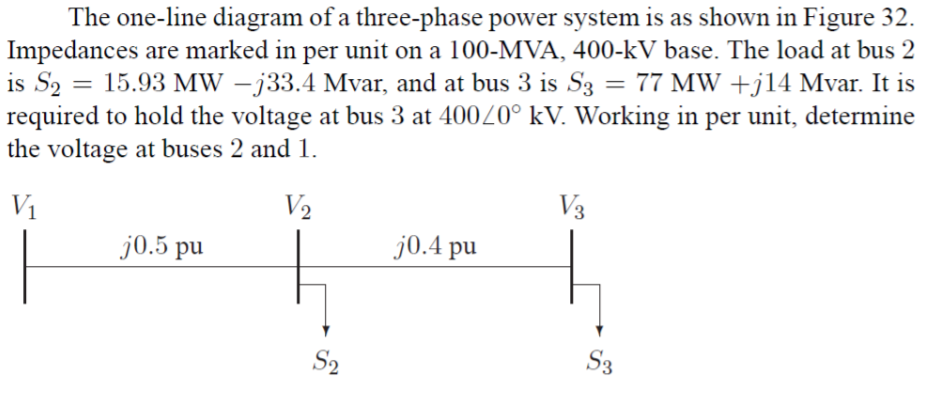# The one-line diagram of a three-phase power system is as shown in Figure 32Impedances are marked in per unit on a 100-MVA, 400-kV base. The load at bus 2is S2--15.93 MW-J33.4 Mvar, and at bus 3 İs S3-77 MN +jl4 Mvar. It isrequired to hold the voltage at bus 3 at 4000° kV. Working in per unit, determinethe voltage at buses 2 andV1V2V3j0.5 puj0.4 pu3

Question
1067 views

The one-line diagram of a three-phase power system is as shown in the figure attached. Impedances are marked in per unit on a 100-MVA, 400-kv base. The load at bus 2 is S(sub 2) = 15.93 MW - j33.4 Mvar, and bus 3 is S(sub 3) = 77MW + j14 Mvar. I tis required to hold the voltage at bus 3 at 400 angle 0 degrees kV. Working in per unit, determine the voltage at buses 2 and 1.help_outlineImage TranscriptioncloseThe one-line diagram of a three-phase power system is as shown in Figure 32 Impedances are marked in per unit on a 100-MVA, 400-kV base. The load at bus 2 is S2--15.93 MW-J33.4 Mvar, and at bus 3 İs S3-77 MN +jl4 Mvar. It is required to hold the voltage at bus 3 at 4000° kV. Working in per unit, determine the voltage at buses 2 and V1 V2 V3 j0.5 pu j0.4 pu 3 fullscreen
check_circle

Step 1

The load at bus is S2=15.93 MW-j33.4 Mvar

First converting all the values in per-unit system

The load in pu, therefore S2=0.1593-j0.334 pu

S3=77 MW+j14 Mvar

In pu its value is S3=0.770+j0.140 pu and also calculating the current flowing into it its calculation is shown on the board:

Step 2

Now calculating the line-line voltage at bus 2 its calculation is shown on the board and also converting the pu value into kV

Therefore, the voltage at bus 2 is 440 kV

Step 3

First calculating the current flowing I2 which is shown on the board

Current flowing from 1 to 2 is...

### Want to see the full answer?

See Solution

#### Want to see this answer and more?

Solutions are written by subject experts who are available 24/7. Questions are typically answered within 1 hour.*

See Solution
*Response times may vary by subject and question.
Tagged in

### Electrical Engineering